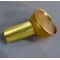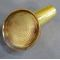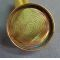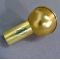##### The Japanese Bonsai specialist
Direct order Contact Help / Services Newsletter Pay in 3 times without charges, from 135 euros in your shopping cart (France residents only)# Nozzle for 4/6-litre watering can

› Bonsai tools › Watering equipment › Copper watering canref. : 7238

21,00

Available quantity : 2Order

###### Description

Made in Japan, this angled copper nozzle is used by Japanese bonsai experts. It is 35 mm and has an interior mouth diameter of 16 mm and 18mm rimmed outside. This matches our Japanese copper 4/6-litre watering cans. The holes are pierced with a lazer resulting in an even and regular jet of water more appropriate for bonsai.

#watering 5.8 #copper 4.6 #nozzle 3.6 #litre 3.5 #japanese 2.8 #bonsai 2.6 #this 2.4 #appropriate 2.1 #resulting 1.9 #diameter 1.8

Formule
(( ROUND((CHAR_LENGTH(b.article_nom)-CHAR_LENGTH(REPLACE(b.article_nom, 'watering', '')))/LENGTH('watering')) + ROUND((CHAR_LENGTH(b.article_description)-CHAR_LENGTH(REPLACE(b.article_description, 'watering', '')))/LENGTH('watering')) ) * 3.8) + (( ROUND((CHAR_LENGTH(b.article_nom)-CHAR_LENGTH(REPLACE(b.article_nom, 'nozzle', '')))/LENGTH('nozzle')) + ROUND((CHAR_LENGTH(b.article_description)-CHAR_LENGTH(REPLACE(b.article_description, 'nozzle', '')))/LENGTH('nozzle')) ) * 3.6) + (( ROUND((CHAR_LENGTH(b.article_nom)-CHAR_LENGTH(REPLACE(b.article_nom, 'litre', '')))/LENGTH('litre')) + ROUND((CHAR_LENGTH(b.article_description)-CHAR_LENGTH(REPLACE(b.article_description, 'litre', '')))/LENGTH('litre')) ) * 3.5) + (( ROUND((CHAR_LENGTH(b.article_nom)-CHAR_LENGTH(REPLACE(b.article_nom, 'japanese', '')))/LENGTH('japanese')) + ROUND((CHAR_LENGTH(b.article_description)-CHAR_LENGTH(REPLACE(b.article_description, 'japanese', '')))/LENGTH('japanese')) ) * 2.8) + (( ROUND((CHAR_LENGTH(b.article_nom)-CHAR_LENGTH(REPLACE(b.article_nom, 'bonsai', '')))/LENGTH('bonsai')) + ROUND((CHAR_LENGTH(b.article_description)-CHAR_LENGTH(REPLACE(b.article_description, 'bonsai', '')))/LENGTH('bonsai')) ) * 2.6) + (( ROUND((CHAR_LENGTH(b.article_nom)-CHAR_LENGTH(REPLACE(b.article_nom, 'copper', '')))/LENGTH('copper')) + ROUND((CHAR_LENGTH(b.article_description)-CHAR_LENGTH(REPLACE(b.article_description, 'copper', '')))/LENGTH('copper')) ) * 2.6) + (( ROUND((CHAR_LENGTH(b.article_nom)-CHAR_LENGTH(REPLACE(b.article_nom, 'this', '')))/LENGTH('this')) + ROUND((CHAR_LENGTH(b.article_description)-CHAR_LENGTH(REPLACE(b.article_description, 'this', '')))/LENGTH('this')) ) * 2.4) + (( ROUND((CHAR_LENGTH(b.article_nom)-CHAR_LENGTH(REPLACE(b.article_nom, 'appropriate', '')))/LENGTH('appropriate')) + ROUND((CHAR_LENGTH(b.article_description)-CHAR_LENGTH(REPLACE(b.article_description, 'appropriate', '')))/LENGTH('appropriate')) ) * 2.1) + (( ROUND((CHAR_LENGTH(b.article_nom)-CHAR_LENGTH(REPLACE(b.article_nom, 'resulting', '')))/LENGTH('resulting')) + ROUND((CHAR_LENGTH(b.article_description)-CHAR_LENGTH(REPLACE(b.article_description, 'resulting', '')))/LENGTH('resulting')) ) * 1.9) + (( ROUND((CHAR_LENGTH(b.article_nom)-CHAR_LENGTH(REPLACE(b.article_nom, 'diameter', '')))/LENGTH('diameter')) + ROUND((CHAR_LENGTH(b.article_description)-CHAR_LENGTH(REPLACE(b.article_description, 'diameter', '')))/LENGTH('diameter')) ) * 1.8)

## Secure payment## Delivery

Our logistic partners :